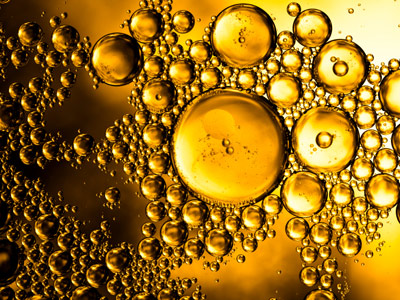I think that as the pH increases, the number of bubbles given off will decrease.

# Unit 4 - Causal or Association Relationship of Variables

Variables are necessary parts of any experiment. In this GCSE Biology quiz we examine both the association relationship (e.g. tall people tend to be heavier, so height and weight are associated) and the causal relationship (e.g. cold temperatures extend food's shelf life, therefore cold temperatures are a cause of extended shelf life) of variables.

Variables are factors in an investigation or experiment which can be changed. They can be changed directly by you, as a result of changes you make during an experiment, or by external factors. In any investigation or experiment there should be one variable that is being changed, one variable that is being measured and the rest should be kept the same. If that is not done, then the investigation or experiment will not be a fair test and the results will not be reliable.

The variables in an investigation or experiment can be classified as independent, dependent or control. The independent variable is the key variable and is the one that you change. The dependent variable is the one that you measure in order to see how the changes of the independent variable affect it. All other variables are classified as control variables and they need to be kept constant. In biology, this can sometimes be very difficult as biology experiments can be spread over several days. In cases like this, where control variables are out of your control (e.g. the amount of cloud), they need to be monitored and noted.

Once you have the results, they need to be processed and interpreted to give a valid conclusion. This is often done by using the appropriate type of graph or chart which can help you to spot any relationships between the variables. A causal relationship means that it is the changes of the key independent variable, X, causing the changes we measure in Y. An association relationship is a correlation between two variables but does not mean that X causes the change in Y. When carrying out complex experiments in biology, it can be difficult to decide whether an effect is causal or an association because of changes in the control variables that are outside the control of the experimenters.

1.
What is an independent variable in a scientific experiment?
A factor which never changes
A factor which changes
A factor whose values are changed or selected by the investigator
A factor which must always be kept the same
It can also be referred to as the key variable
2.
Which is the key independent variable of the hypothesis "I think that as the temperature increases, the rate of bubbles given off will increase due to the increased number of collisions of particles."?
Collisions
Number of bubbles
Rate
Temperature
In this experiment you would be changing the temperature and observing the number of bubbles given off. The variable that you will be changing is the independent variable
3.
Causal implies that...
X caused Y to increase
An unknown factor caused Y to increase
Z was involved
Other factors were involved
When a correlation is spotted, it is then time to see if scientific theory can be used to explain it. If it can't then the correlation may not be causal. Scientists working at the 'cutting edge' of their subject may have to develop new theories to explain the correlation. This is often how new discoveries are made
4.
The dependent variable is...
the factor we change
the factor we control
the factor we measure
the X factor
It is called this because its values depend on the values chosen for the independent variable
5.
An association is not the same thing as a causal relationship because...
causal means one thing has definitely influenced the outcome
causal means other factors could have caused the outcome
association means they are connected by lots of factors
association is not relevant
Sometimes the correlation is associative because one of the control variables has not been identified and controlled
6.
How many different types of variables are there?
One
Two
Three
Four
Dependent, independent and control
7.
Directly proportional means that...
as X increases, Y increases in proportion giving a straight line graph
as X increases, Y decreases
as X increases, Y increases a small amount
As X increases, Y increases greatly
On a scatter graph, if the line of best fit is straight, you have a relationship where the dependent variable is directly proportional to the independent variable. In other words, whenever you double variable X, variable Y will double too
8.
The key independent variable is...
the factor we change
the factor we keep the same
the factor we measure
the factor we ignore
The key independent variable is the factor we are studying in our investigation and is the basis for our hypothesis
9.
As X increases, Y increases demonstrates a...
negative correlation
positive correlation
causal relationship
association relationship
We have no way of knowing if X caused Y to increase, but if X increased and Y also increased, there is a positive correlation
10.
What is the dependent variable in the prediction "I think that as the pH increases, the number of bubbles given off will decrease."?
pH
Decrease
Increase
Number of bubbles
The dependent variable is the one you measure in the experiment